## Tutorial: Calculate time of concentration (Kirpich)

### 6. Calculate the time of concentration in PyQGIS

Because there's no easy way to calculate the Kirpich equation with the field calculator in the attribute table, we're going to develop a PyQGIS script in this section to implement the Kirpich equation

The Kirpich equation:

tc = k * (dx ** const1) * (S ** const2)

Where:

tc = time of concentration in minutes
k = 0.0195 to convert to SI units
dx = distance between the first and last node in meters
S = the elevation difference between the first and last node in m/m
const1 = 0.77
const2 = -0.385

1. In the main menu go to Plugins | Python ConsoleNow you'll see the Python console appearing at the bottom of the screen.

2. Click the Show Editor icon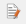.

This opens the editor where we can write code. A free tutorial to start learning PyQGIS can be found here.

3. Write the code from the screenshot below. The lines with # give and explanation. Alternatively you can download the script from GitHub.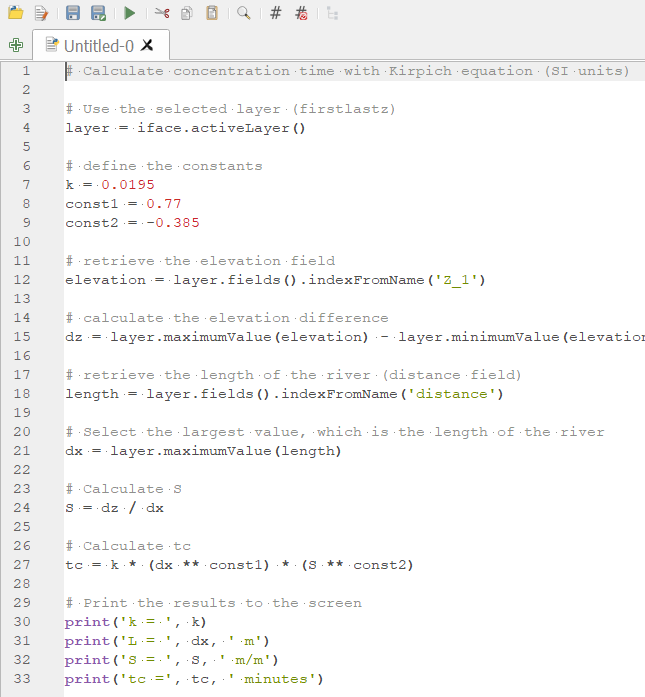4. Click the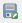button and save the script as Kirpich.py

5. Click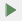to run the script

You'll see the results in the Python console: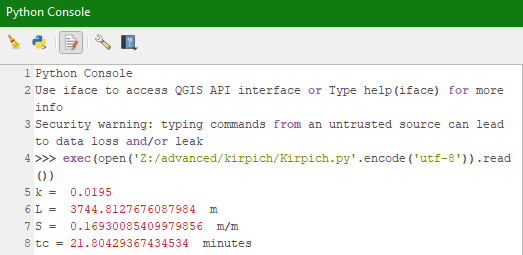The concentration time is 21.8 minutes.